# Sophie Germain prime

(diff) ← Older revision | Latest revision (diff) | Newer revision → (diff)
This article defines a property that can be evaluated for a prime number. In other words, every prime number either satisfies this property or does not satisfy this property.
View other properties of prime numbers | View other properties of natural numbers

## Definition

A Sophie Germain prime is a prime numbersuch that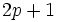is also prime. The corresponding primeis termed a safe prime.

## Occurrence

### Initial values

The first few Sophie Germain primes are:

2, 3, 5, 11, 23, 29, 41, 53, 83, 89, [SHOW MORE]View list on OEIS

The first few primes that are not Sophie Germain primes are: 7, 13, 17, 19, 31.

### Density in primes

CutoffNumber of primesNumber of Sophie Germain primesProportion of primes that are Sophie Germain primes Number of Sophie Germain primes divided by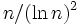10 4 3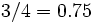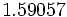100 25 10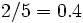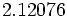1000 168 37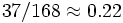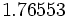### Infinitude conjecture

Further information: Infinitude conjecture for Sophie Germain primes

It is conjectured that there are infinitely many Sophie Germain primes (or equivalently, that there are infinitely many safe primes). It is also conjectured that the number of Sophie Germain primes less than or equal tois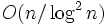, in other words, there exist constantssuch that the following holds for all sufficiently large:.

where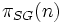denotes the number of Sophie Germain primes less than or equal to.

Other related conjectures to the existence of Sophie Germain primes are:

### Infinitude of complement

Most primes are not Sophie Germain primes. It is rather easy to see that the number of primes that are not Sophie Germain primes is infinite: for instance, there are infinitely many primes that aremodulo, and none of them can be a Sophie Germain prime.

## Facts

While there are not too many interesting facts about the structure of Sophie Germain primes per se, the structure of the corresponding safe primes is very interesting:

## Testing

The ID of the sequence in the Online Encyclopedia of Integer Sequences is A005384